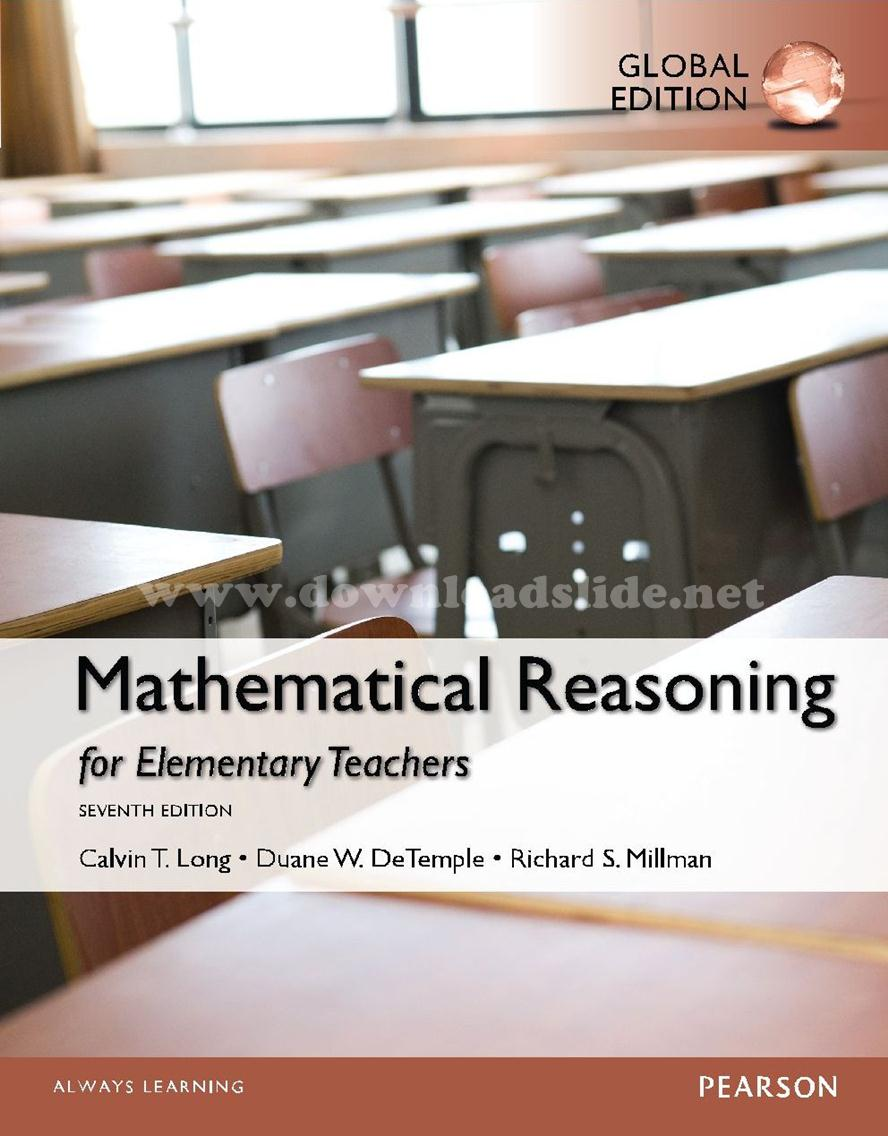### Ebook Mathematical Reasoning 7e by Long, DeTemple, Millman (Global Edition)Mathematical Reasoning for Elementary Teachers
Global Edition, 7th Edition
Calvin T. Long, Duane W. DeTemple, Richard S. Millman

Table of Content:
1. Thinking Critically
2. Sets and Whole Numbers
3. Numeration and Computation
4. Number Theory
5. Integers
6. Fractions and Rational Numbers
7. Decimals, Real Numbers, and Proportional Reasoning
8. Algebraic Reasoning, Graphing, and Connections with Geometry
9. Geometric Figures
10. Measurement: Length, Area, and Volume
11. Transformations, Symmetries, and Tilings
12. Congruence, Constructions, and Similarity
13. Statistics: The Interpretation of Data
14. Probability Probability, and Simulations